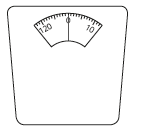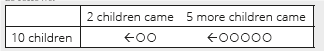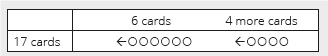# Lesson Notes By Weeks and Term - Primary 2

Problem solving

TERM: 2nd Term

WEEK: 9

CLASS: Primary 2

AGE: 7 years

DURATION: 5 periods of 40 minutes each

DATE:

SUBJECT: Mathematics

TOPIC: Problem solving

SPECIFIC OBJECTIVES: At the end of the lesson, the pupils should be able to

1. Solve word problems which involve two increases which need to be calculated one at a time.
2. solve word problems with two increases by making a ten.
3. Solve simple addition and subtraction problems involving mass.

INSTRUCTIONAL TECHNIQUES: Explanation, question and answer, demonstration, practical

INSTRUCTIONAL MATERIALS: Bottle tops

PERIOD 1: Assessment

 PRESENTATION TEACHER’S ACTIVITY PUPIL’S ACTIVITY STEP 1ORALASSESSMENTS The teacher asks questions on mental maths treated so far Pupils respond and participate STEP 2DISCUSSION The teacher discusses all the methods used by some learners in the oral assessments(some of the questions are solved on the board by the learners) and addresses any misconceptions that may have risen Pupils pay attention and participate STEP 3WRITTEN ASSESSMENTS 1a What mass reading is shown on this bathroom scale? _______________b Is anyone standing on the scale? Circle: yes/noc How do you know? _______________________2 20 kg + 11 kg = ___3 21 kg – 14 kg = ___4 Arrange from lightest to heaviest: 8 kg, 5 kg, 2 kg, and 10 kg.______________5 Solve the problems:a Sam’s mass is 21 kg. Steve’s mass is 20 kg. How many kilograms is their total mass? b Mom buys 12 kg potatoes. Dad buys 5 kg potatoes. How many kilograms more did Mom buy than Dad? Pupils attempt their class work STEP 4SUMMARY The teacher marks the written assessments, corrects were necessary and commends the pupils

PERIOD 2: Problem solving (1)

 PRESENTATION TEACHER’S ACTIVITY PUPIL’S ACTIVITY STEP 1MENTAL MATHS The teacher begins the lesson with some mental calculationswhich is moreCalculate1 80 + 3 =  2 70 + 5 =  3 90 + _ =  944 _ + 70 = 75  5 _+ 9 = 696 10 + 90=7 50 + 8 =8 _ + 90 = 979 80 + _ =8210 _ + 40 = 46 Pupils respond and participate STEP 2CONCEPTDEVELOPMENT The teacher• Writes the following word problem on the board. There are 10 children in a room.2 children came in andthen 5 more came in.How many children are there now? • The word problem must be written on four lines as shown above to assist learners to identify the critical information/numbers needed to solve the problem.• Reads the problem.• Lets learners read the problem until they read it fluently.• Underlines the numbers, 10, 2 and 5.• Underlines the question (How many children are there now?) with a wavy line.• Lets learners manipulate bottle tops to represent the story.• Then, let them draw circles in their classwork book (with words explaining it) as follows.• Draws a diagram as above.This is a special case diagram since two numbers are added. This diagram helps learners focus on the numbers to add to the first number. Hence, teachers can draw it and learners copy it.• Lets the learners draw the diagram.• Lets learners determine the operation from the bar diagram and write the number sentence.• Lets learners present their number sentence and confirm with the class the correct number sentence.• Lets the learners solve the number sentence (10 + 2 + 5 = 17).• Asks: How did you do the calculation? (a. 10 + 2 = 12, then 12 + 5 = 17 or b. 2 + 5 = 7,10 + 7 = 17).If all the learners answered in the answer a, ask the following questions.How many children increased in total? (2 + 5 = 7)How many is the total in that case? (10 + 7 = 17)• Discusses the differences between the 2 methods with the learners. Let them explain which method they prefer and why.• Asks: What is the answer for the word problem? (There are 17 children now.)Learners have to answer with unit, 17 children. CLASS ACTIVITYThe teacher• Writes the following word problem on the board: There were 12 birds in the park.7 birds flew in andthen another 3 came. How many birds are there now?• Using the steps from activity 1 solves this word problem with the learners.• (12 + 7 + 3 = 19 + 3 = 22 or 12 + 7 + 3 = 12 + 10 = 22, 22 birds.) Pupils pay attention and participate STEP 3CLASS-WORK Note: Do not erase the workings for Activity 1 and 2. The learners will need these to guide them with their classwork. In this activity learners as asked to solve the questions using twodifferent methods. They could add the numbers in the order that they are written or they could add them by selecting a different order to add the numbers. Add these numbers:1 Add them in the order in which they are written.2 Add them by writing them in a different order.a 10 + 2 + 4 =  b 10 + 6 + 3 =c 11 + 4 + 3 =  d 12 + 2 + 3 =e 13 + 3 + 3 =  f 12 + 1 + 5 = Pupils attempt their class work STEP 4HOME-WORK Add these numbers:1 Add them in the order in which they are written.2 Add them by writing them in a different order.a 10 + 2 + 1 =b 12 + 3 + 2 =c 14 + 2 + 2 =  d 15 + 3 + 1 = The pupils writes it in their homework book STEP 5SUMMARY The teacher summarizes by reminding the pupils that in this lesson we have learnt to solve addition and subtraction word problems through increasingthe terms. She marks their class works, makes corrections where necessary and commends them positively

PERIOD 3: Problem solving (2)

 PRESENTATION TEACHER’S ACTIVITY PUPIL’S ACTIVITY STEP 1MENTAL MATHS The teacher begins the lesson with some mental calculationsCalculate1 13 + 10 =  2 15 + 30 =  3 36 + 20 =  4 35 + 50 =  5 28 + 70 =  6 30 + 14 =7 50 + 23 =8 40 + 26 =9 50 + 34 =10 30 + 65 = Pupils respond and participate STEP 2CONCEPTDEVELOPMENT The teacher• Writes the following word problem on the board. Sophie had 17 cards.She bought 6 cards yesterday and4 cards today.How many cards does she have now? The word problem must be written on four lines as shown above to assist learners toidentify the critical information/numbers needed to solve the problem.• Reads the problem.• Lets learners read the problem until they read it fluently.• Underlines the numbers, 17, 6 and 4.• Underlines the question (How many cards does she have now?) with a wavy line.• Lets learners manipulate bottle tops to represent the story.Then, let them draw circles in their classwork book (with words explaining it)as follows.• Draws a diagram as above.This is a special case diagram. This diagram helps learners focus on the numbers to add to the first number. Hence, teachers can draw it and learners copy it.• Lets the learners draw the diagram.• Lets learners determine the operation from the bar diagram and write the numbersentence.• Lets learners present their number sentence and confirm with the class the correct number sentence.• Lets the learners solve the number sentence (17 + 6 + 4 = 27).• Asks: How did you do the calculation? (a. 17 + 6 = 23, then 23 + 4 = 27 or b. 6 + 4 = 10, 17 + 10 = 27).If all the learners answered in the answer a, ask the following questions.How many cards increased in total? (6 + 4 = 10)How many is the total in that case? (17 + 10 =27)• Discusses the differences between the 2 methods with the learners. Let them explainwhich method they prefer and why.Learners are expected to find that adding 10 (answer b) is easier than carrying (answer a). If they don’t agree, learners need to practise adding multiples of 10 using base ten kits.• Asks: What is the answer for the word problem? (There are 27 cards now.) Learners have to answer with the unit, 27 cards. ACTIVITY IIThe teacher• Writes the following word problem on the board:Thandi had 12 marbles.He bought 8 marbles yesterday.Then he bought another 5 today.How many marbles does he have now? • Using the steps from activity 1 solve this word problem with the learners.• Discuss: This time, adding from left in order is easier because if you add 8 + 5 first, it includes addition with carrying and then, adding 2-digit numbers. Rather, add 12 + 8 first to make 20 which is a multiple of 10.12 + 8 + 5 = 20 + 5 = 25 or (8 + 5 = 13, 12 + 13 = 25), 25 marbles. Pupils pay attention and participate STEP 3CLASS-WORK Note: Do not erase the workings for Activity 1 and 2. The learners will need these to guide them with their classwork.1 Add these numbers. Think about how to pair the numbers when you add.a 15 + 6 + 4 =b 14 + 6 + 7 =c 24 + 6 + 9 =d 34 + 8 + 2 =e 43 + 7 + 4 =f 54 + 8 + 2 =2 Solve the problem.There are 18 chickens on the farm.The farmer buys another 9 andthen another 1 chicken.How many chickens does the farmer have altogether? Pupils attempt their class work STEP 4HOME-WORK Solve the questions. Think about how to pair the numbers when you add.a 13 + 8 + 2 =b 15 + 5 + 8 =c 24 + 6 + 4 =d 47 + 8 + 2 = The pupils writes it in their homework book STEP 5SUMMARY The teacher summarizes by reminding the pupils that in today’s lesson we have learnt have learnt to solve word problems by choosing the most efficient approach.She marks their class works, makes corrections where necessary and commends them positively

PERIOD 4: Problem solving(3)

 PRESENTATION TEACHER’S ACTIVITY PUPIL’S ACTIVITY STEP 1MENTAL MATHS The teacher begins the lesson with some mental calculationsCalculate1 12 + 4=  2 15 – 2=3 14 – 3 =  4 12+ 6 =  5 14 + 3=  6 17 – 5 =7 13 + 6 =  8 16 – 5 =9 12 +7 =  10 18 – 6 = Pupils respond and participate STEP 2CONCEPTDEVELOPMENT The teacher• Write the following word problem on the board.There were 15 passengers on the bus.6 passengers got on and2 passengers got off at the next bus stop.How many passengers are there on the bus now?The word problem must be written on four lines as shown above to assist learners to identify the critical information/numbers needed to solve the problem.• Reads the problem.• Lets learners read the problem until they read it fluently.• Underlines the numbers, 15, 6 and 2.• Underlines the question (How many passengers are there on the bus now?) with a wavy line.• Lets learners manipulate bottle tops to represent the story.• Then, let them draw circles in their classwork book (with words explaining it) as follows.• Draws a diagram as above.This is a special case diagram. This diagram helps learners focus on the numbers to add to the first number. Hence, teachers can draw it and learners copy it.• Lets the learners draw the diagram.• Lets learners determine the operation from the bar diagram and write the number sentence.• Lets learners present their number sentence and confirm with the class the correct number sentence.• Lets the learners solve the number sentence (15 + 6 – 2 = 19).• Asks: How did you do the calculation? (a. 15 + 6 = 21, then 21 – 2 = 19 or b. 6 – 2 = 4,15 + 4 = 19).If all the learners answered in the answer a, ask the following questions.By how many did the passengers increase in total? (6 – 2 = 4)How many is the total in that case? (15 + 4 =19)• Discusses the differences between the 2 methods with the learners. Let them explain which method they prefer and why.Subtracting the third number from the second number first is easier because if we add, then subtract, we have to calculate addition with carrying and subtraction with borrowing.• Asks: What is the answer for the word problem? (There are 19 passengers now).Learners have to answer with unit, 19 passengers. CLASS ACTIVITYThe teacher• Writes the following word problem on the board: Tim had 17 sweets.His mom gave him 5 sweets.Then he ate 4 sweets.How many sweets does Tim have left? • Using the steps from activity 1 solve this word problem with the learners.• (17 + 5 – 4 = 22 – 4 = 18) or 17 + 5 – 4 = ,5 – 4 = 1, 17 + 1 = 18, 18 sweets. Pupils pay attention and participate STEP 3CLASS-WORK Note: Do not erase the workings for Activity 1 and 2. The learners will need these to guide them with their classwork.1 Solve the questions. Think about how to pair the numbers when you add.a 16 + 5 – 4 =b 15 + 8 – 4 =c 24 + 7 – 5 =  d 34 + 9 – 6 =e 46 + 8 – 5 =  f 57 + 7 – 6 =  2 Solve the problem.There were 19 cars in the parking lot.5 more cars parked.Then 4 cars left.How many cars are left? Pupils attempt their class work STEP 4HOME-WORK Solve the questions. Think about how to pair the numbers when you add.a 17 + 4 – 2 =b 14 + 7 – 3 =c 23 + 8 – 6 =d 43 + 9 – 8 = The pupils writes it in their homework book STEP 5SUMMARY The teacher summarizes by reminding the pupils that in today’s lesson we have solved addition and subtraction word problems using different methods. She marks their class works, makes corrections where necessary and commends them positively

PERIOD 5: Weekly Test/consolidations

TEACHER’S ACTIVITY: The teacher revises all the concepts treated from period 1-4 and gives the pupils follow through exercises, quiz and tests. She marks the exercises, makes corrections and commends the pupils positively.

PUPIL’S ACTIVITY: The pupils work on the worksheets and exercises give by the teacher individually

CONSOLIDATION

Read the problems below. Solve them using 2 different methods.

1 I picked 7 apples on Monday.

I picked 3 apples on Tuesday and

5 more apples on Wednesday.

How many apples did I pick altogether?

2 There were 14 children swimming in the pool.

7 children arrive and

then another 3 arrive.

How many children are there altogether swimming in the pool?

3 Maria has 16 crayons.

Her mom buys her another 5 crayons.

She loses 4 of the crayons.

How many crayons does she have left?

4 There were 14 children in the classroom.

6 children arrived in the classroom and

then another 3.

How many children are there now?

5 There were 23 cows in the field.

6 more cows arrived in the field and

then another 4.

How many cows are there now?

6 There were 12 pigs in the field.

6 more pigs came to the field and

then 5 pigs left.

How many pigs are left in the field?